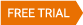# Profit Maximisation Models – Part 2# Profit Maximisation Models

We recently introduced Profit Maximisation Models in a previous article. If you missed the first part you can read it here.

Now lets delve into some other Profit Maximisation Models.

## Kelly Criterion

The Kelly Criterion is probably the most practical and ‘institutional’ way to maximise returns of a system with a positive expectancy. It was developed by John Kelly, who worked for AT&T’s Bell Laboratory to assist with its long distance telephone signal noise issues. Since the 1950’s it has been the cornerstone technique for horse and blackjack betting systems so it’s a worthy consideration when applied to trading systems as well. The Kelly Criterion is a little more scientific that the Fixed Ratio approach and attempts to define the optimal bet size to grow an account.

The formula is:

Kelly % = W – [(1 – W) / R]

Where,
W = Win%
R = W/L Ratio

Using some data from a random mean reversion strategy, where the Win% was 63.2% and the W/L Ratio was 0.963, the Kelly % is 24.99%, meaning we should bet 24.99% of account equity on each trade.

Yikes!

The usual course of action is to use a fraction of the Kelly %, usually 50%. Even so, betting 12.5% of equity on each trade is still very high and will lead to significant account volatility.

## Optimal-f and Secure-f

Optimal-f was designed by Ralph Vince to define the optimal bet size for maximum equity growth. It is theoretical because eventually a single losing trade will blow the account out.

Optimal-f calculates the bet size that maximises the rate of return for a given series of trades. While this sounds like a good idea, in practice relying on the historical sequence of trades is risky in that the sequence of profits and losses in the future may be less favorable than what was encountered historically. As a result, the drawdowns in the future could be much larger than predicted by the historical sequence of trades.

The equation is complex because we need a series of trades to compute the outcome:

Calculate the value of f that maximises: W = (1 + f T1/L)(1 + f T2/L)…(1 + f Tn/L)

Where,
W is the optimal number of shares to trade
f = multiple determined from historical trades (between 0 and 1)
Tn is the trading profit or loss for the n-th trade (example: T1 = \$123, T2 = -\$456, etc.)
L is the maximum loss per trade (example: L = \$100)

As can be seen the calculations can become quite onerous.

Research has even extended to a more conservative variant known as Secure-f although the calculations become even more complex.

There are many different ways to grow the account. Using a linear method such as Fixed Fractional position sizing will do it, albeit slowly and surely.

The key with growing the account is that you need to take on more risk, and taking on more risk means exposing oneself to a higher drawdown. Drawdowns on paper are easy to digest, but in real life there is a lot more emotional baggage to be carried. So first and foremost think long and hard about how much drawdown you can really withstand.

##### PS: This information is a small part of the material offered in the Trading System Mentor Course . There are currently several spots available in 2020 for the right candidate.